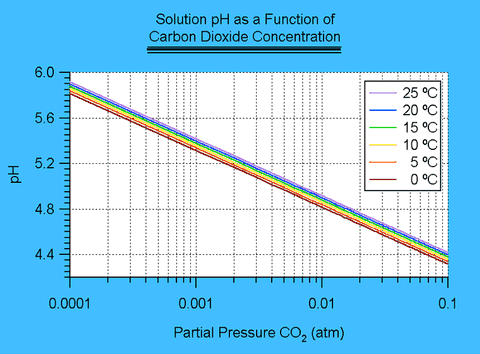## Appendix IIThe Effect of Carbon Dioxide on pH

Carbon dioxide (CO2) is an acid anhydride, which means that when it is dissolved in water it lowers the pH of the solution. In fact pure rainwater has a pH of 5.6 because of the 360 ppm atmospheric concentration of CO2. During World War II, atmospheric concentrations of CO2 were closer to 330 ppm, but that is not an important difference for the purposes addressed here. One can think of the process as having three steps all of which involve a chemical equilibrium. The first step is the dissolution of CO2 in water; its equilibrium constant is the Henry's Law constant of CO2, Khc:

CO2 + H2O <=> H2CO3

The second step is the acid base reaction between carbonic acid and water; its equilibrium constant is denoted here as Kc1:

H2CO3 + H2O <=> H3O+ + HCO3-

The third step is reaction between the bicarbonate ion (HCO3-) and water whose equilibrium constant is here denoted as Kc2:

HCO3- + H2O <=> H3O+ + CO32-

Also, one needs to consider the autoprotolysis of water:

2H2O <=> H3O+ + OH-

In aqueous solution, one can treat the concentration of water as a constant and derive a constant for the equilibrium, Kw. These constants and their associated enthalpies are reported in Seinfeld. 1 Here is a table of the relevant quantities:

Table II.1: Equilibrium Constants and Associated Enthalpies
for the Absorption of Carbon Dioxide by Water.
ConstantValueEnthalpy at 298 K
(kcal/mole)
Khc3.4 x 10-2-4.846
Kc14.283 x 10-71.825
Kc24.687 x 10-113.55
Kw 1.008 x 10-1413.345

The slight temperature dependence of these constants can be accounted for by means of the enthalpies given. The pH of a solution is defined as -log[H+] where [H+] is the concentration of hyronium ions in moles/liter. From the information given it is possible to derive a cubic equation expressing the relationship between [H+] and the partial pressure of CO2, PCO2. This equation is also presented in Seinfeld: 1

[H+]3 - (Kw+KhcKc1 PCO2)[H+]-2+KhcKc1 Kc2PCO2=0

I solved this equation by means of a simple computer program employing a rootfinding method similar to Newton's method. 2 The results are shown in figure II.1.

### Figure II.1